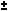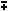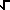Dave's Math Tables: Polynomial Identities(Math | Algebra | Polynomials)

(a+b) 2 = a 2 + 2ab + b 2

(a+b)(c+d) = ac + ad + bc + bd

a 2 - b 2 = (a+b)(a-b) (Difference of squares)

a 3b 3 = (ab)(a 2ab + b 2) (Sum and Difference of Cubes)

x 2 + (a+b)x + ab = (x + a)(x + b)

if ax 2 + bx + c = 0 then x = ( -b(b 2 - 4ac) ) / 2a (Quadratic Formula)